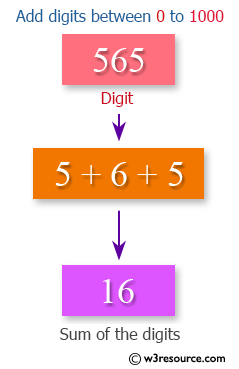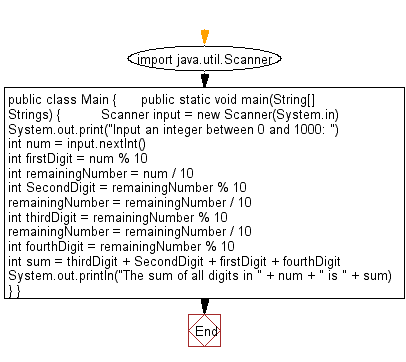﻿ Java exercises: Adds all the digits in the integer between 0 and 1000 - w3resource# Java Data Type Exercises: Adds all the digits in the integer between 0 and 1000

## Java Data Type: Exercise-3 with Solution

Write a Java program that reads an integer between 0 and 1000 and adds all the digits in the integer.

An integer is a number that can be written without a fractional component. For example, 23, 6, 0, and −1245 are integers, while 3.25, ​7 1⁄2, and √3 are not.

Test Data
Input an integer between 0 and 1000: 565Sample Solution:

Java Code:

``````import java.util.Scanner;
public class Main {

public static void main(String[] Strings) {

Scanner input = new Scanner(System.in);

System.out.print("Input an integer between 0 and 1000: ");
int num = input.nextInt();

int firstDigit = num % 10;
int remainingNumber = num / 10;
int SecondDigit = remainingNumber % 10;
remainingNumber = remainingNumber / 10;
int thirdDigit = remainingNumber % 10;
remainingNumber = remainingNumber / 10;
int fourthDigit = remainingNumber % 10;
int sum = thirdDigit + SecondDigit + firstDigit + fourthDigit;
System.out.println("The sum of all digits in " + num + " is " + sum);

}
}
```
```

Sample Output:

```Input an integer between 0 and 1000: 565
The sum of all digits in 565 is 16
```

Flowchart:Java Code Editor:

Improve this sample solution and post your code through Disqus

What is the difficulty level of this exercise?

﻿

## Java: Tips of the Day

Checks if a string is upper case:

```public static boolean isUpperCase(String input) {
return Objects.equals(input, input.toUpperCase());
}
```

Ref: https://bit.ly/39Hpo84